#### 期刊菜单

Low Carbon Optimal Scheduling Method of Integrated Energy System Based on Carbon Emission Analysis
DOI: 10.12677/SG.2022.123010, PDF, 下载: 119  浏览: 464  科研立项经费支持

Abstract: In today’s world, the environmental problems in the world are becoming increasingly prominent, and the development of integrated energy system has become an important means to realize the low-carbon energy industry. Based on this, this paper proposes an integrated energy carbon emission optimization method aiming at carbon reduction. Firstly, the carbon emissions of all kinds of energy in the integrated energy system are analyzed. Secondly, aiming at reducing carbon emissions, the optimization model of carbon emissions of integrated energy is established and the particle swarm algorithm is used to solve the model. Finally, the simulation results show that the proposed method can not only effectively reduce the carbon emissions of the integrated energy system, but also increase the consumption level of wind power and photoelectric energy of the integrated energy system.

1. 引言

2. 综合能源系统各类能源碳排放量分析

1) 燃煤机组碳排放模型

${Q}_{c.j}=\underset{t=1}{\overset{T}{\sum }}\left({\alpha }_{1}{P}_{C.j.t}^{2}+{\beta }_{1}{P}_{C.j.t}+{\lambda }_{1}\right)$ (1)

2) 水电机组碳排放模型

${Q}_{wa.j}=\underset{t=1}{\overset{T}{\sum }}{\lambda }_{wa}{P}_{wa.j.t}$ (2)

3) 风电机组碳排放模型

${Q}_{w.j}=\underset{t=1}{\overset{T}{\sum }}{\lambda }_{w}{P}_{w.j.t}$ (3)

4) 光伏碳排放模型

${Q}_{p.j}=\underset{t=1}{\overset{T}{\sum }}{\lambda }_{p}{P}_{p.j.t}$ (4)

5) 燃气轮机碳排放模型

${Q}_{g.j}=\underset{t=1}{\overset{T}{\sum }}{\lambda }_{g}{P}_{GT.j.t}$ (5)

3. 以降碳为目标的综合能源碳排放优化方法

3.1. 以降碳为目标的综合能源碳排放优化模型

$\mathrm{min}Q=\underset{j=1}{\overset{{N}_{C}}{\sum }}{Q}_{c.j}+\underset{j=1}{\overset{{N}_{G}}{\sum }}{Q}_{g.j}+\underset{j=1}{\overset{{N}_{W}}{\sum }}{Q}_{w.j}+\underset{j=1}{\overset{{N}_{P}}{\sum }}{Q}_{p.j}+\underset{j=1}{\overset{{N}_{wa}}{\sum }}{Q}_{wa.j}$ (6)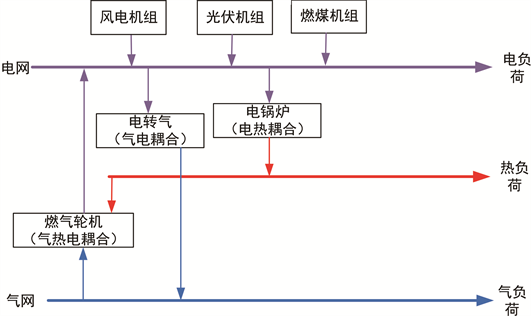Figure 1. Energy hub model diagram

$\mathrm{min}Q=\underset{j=1}{\overset{{N}_{C}}{\sum }}{Q}_{c.j}+\underset{j=1}{\overset{{N}_{G}}{\sum }}{Q}_{g.j}$ (7)

$\mathrm{min}Q=\underset{j=1}{\overset{{N}_{C}}{\sum }}\underset{t=1}{\overset{T}{\sum }}\left({\alpha }_{1}{P}_{C.j.t}^{2}+{\beta }_{1}{P}_{C.j.t}+{\lambda }_{1}\right)+\underset{j=1}{\overset{{N}_{G}}{\sum }}\underset{t=1}{\overset{T}{\sum }}{\lambda }_{g}{P}_{GT.j.t}$ (8)

3.2. 约束条件

3.2.1. 各能源约束

1) 燃煤机组约束

$\left\{\begin{array}{l}{P}_{C.j.\mathrm{min}}\le {P}_{C.j.t}\le {P}_{C.j.\mathrm{max}}\hfill \\ {P}_{C.j.\text{downmax}}\le {P}_{C.j.t}-{P}_{C.j.t-1}\le {P}_{C.j.\text{upmax}}\hfill \end{array}$ (9)

2) 燃气轮机约束

$\left\{\begin{array}{l}{P}_{GT.j.min}\le {P}_{GT.j.t}\le {P}_{GT.j.max}\\ {P}_{GT.j.downmax}\le {P}_{GT.j.t}-{P}_{GT.j.t-1}\le {P}_{GT.j.upmax}\end{array}$ (10)

3) 风电机组约束

$0\le {P}_{W.j.t}\le {P}_{W.j.\mathrm{max}}$ (11)

4) 光伏机组约束

$0\le {P}_{P.j.t}\le {P}_{P.j.\mathrm{max}}$ (12)

5) 水电机组约束

$0\le {P}_{WA.j.t}\le {P}_{WA.j.\mathrm{max}}$ (13)

3.2.2. 综合能源电力平衡约束

$\underset{j=1}{\overset{{N}_{W}}{\sum }}{P}_{W.j.t}+\underset{j=1}{\overset{{N}_{WA}}{\sum }}{P}_{WA.j.t}+\underset{j=1}{\overset{{N}_{P}}{\sum }}{P}_{P.j.t}+\underset{j=1}{\overset{{N}_{c}}{\sum }}{P}_{C.j.t}+\underset{j=1}{\overset{{N}_{G}}{\sum }}{P}_{G.j.t}={P}_{e.t}^{load\text{}}+{P}_{ht}^{load}$ (14)

3.3. 模型求解

1) 设初始种群数为100，各时段风电机组、光伏机组、燃煤机组、燃气轮机、水电机组的发电功率的位置变量为X11-X1T，X21-X2T，X31-X3T……，各时段的机组变化量为V，速度序号为V11-V1T，V21-V2T，V31-V3T……。

2) 对每个粒子进行随机初始化。

3) 计算目标函数值并与上一代的值进行比较，更新个体最优值 ${g}_{j}$ 和全局最优值m。

4) 根据目标函数的适应度更新搜索速度和位置，具体如下：

${V}_{i,j}^{t+1}={V}_{i,j}^{t}+{c}_{1}{r}_{1}\left({P}_{i,j}-{X}_{i,j}^{t}\right)+{c}_{2}{r}_{2}\left({g}_{j}-{X}_{i,j}^{t}\right)$ (15)

5) 判断是否达到最大迭代次数，若达到则结束计算，输出全局最优值。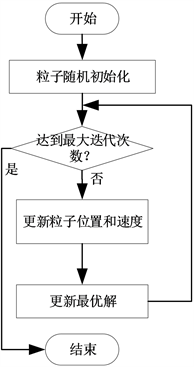Figure 2. Calculation flow chart

4. 算例分析

4.1. 算例概述

1) 该系统包含一座容量为15 MW的风电站W1，一座容量为15 MW的光伏电站P1，一座容量为15 MW的水电站WA1，一座容量为40 MW的燃煤电厂C1和一座容量为40 MW的燃气电厂G1。其中燃气电厂的碳排放强度取450 g/kW∙h，燃煤电厂的碳排放强度取800 g/kW∙h，燃煤机组的最小技术出力为其额定容量的50%，爬坡速率为每个小时上升或下降50%的机组额定容量；燃气轮机的最小出力为额定容量的30%，爬坡速率为每个小时上升或下降100%的机组额定容量；水电机组的爬坡速率为每个小时上升或下降100%的机组额定容量；

2) 系统电热负荷预测图如图3所示：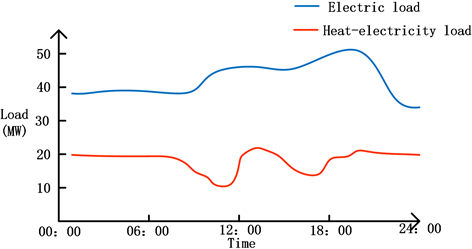Figure 3. Electric heating load prediction curve of integrated energy system

3) 风、光、水电预测出力如图4所示：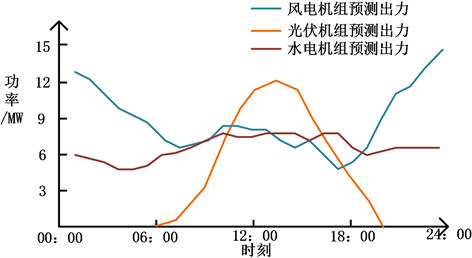Figure 4. Forecast value of output of wind, photovoltaic and hydro-power in 24 h

4.2. 仿真结果分析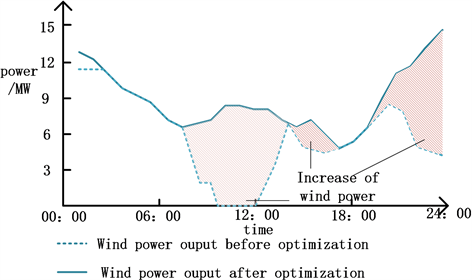Figure 5. Wind power planned output before and after optimizationFigure 6. Photovoltaic planned output before and after optimization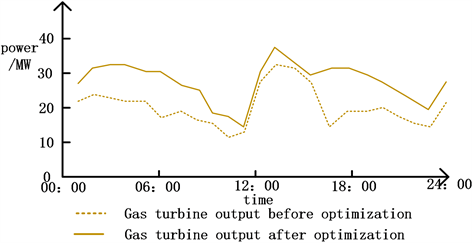Figure 7. Gas turbine planned output before and after optimization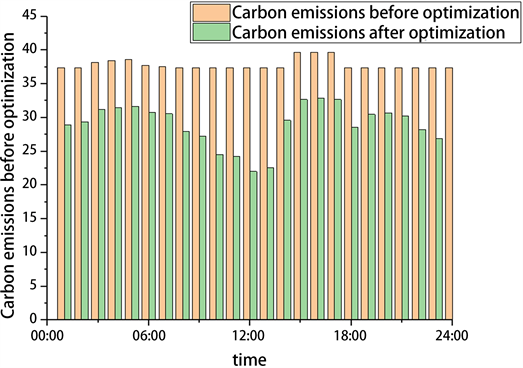Figure 8. Carbon emissions in each period before and after optimizationTable 1. Running results before and after optimization

5. 结论

1) 以降碳为目标的碳排放优化方法可有效降低综合能源系统碳排放量。

2) 该方法有效提高了综合能源系统风电光伏的消纳能力。

  米剑锋, 马晓芳. 中国CCUS技术发展趋势分析[J]. 中国电机工程学报, 2019, 39(9): 2537-2544.  李更丰, 黄玉雄, 别朝红, 安佳坤, 孙思源, 邱起瑞, 高晓松, 彭钰茗, 雷宇骁. 综合能源系统运行可靠性评估综述及展望[J]. 电力自动化设备, 2019, 39(8): 12-21.  崔杨, 曾鹏, 仲悟之, 崔文利, 赵钰婷. 考虑阶梯式碳交易的电-气-热综合能源系统低碳经济调度[J]. 电力自动化设备, 2021, 41(3): 10-17.  邹晨露, 崔雪, 周斌, 高健, 刘洋. 低碳环境下计及柔性负荷和电锅炉的电热联合系统优化调度[J]. 电测与仪表, 2019, 56(18): 34-40+56.  车泉辉, 吴耀武, 祝志刚, 娄素华. 基于碳交易的含大规模光伏发电系统复合储能优化调度[J]. 电力系统自动化, 2019, 43(3): 76-82+154.  史佳琪, 胡浩, 张建华. 计及多个独立运营商的综合能源系统分布式低碳经济调度[J]. 电网技术, 2019, 43(1): 127-136.  秦婷, 刘怀东, 王锦桥, 冯志强, 方伟. 基于碳交易的电-热-气综合能源系统低碳经济调度[J]. 电力系统自动化, 2018, 42(14): 8-13+22.  李保卫, 胡泽春, 宋永华, 方晓松, 杨俊. 用户侧电力碳排放强度的评估原则与模型[J]. 电网技术, 2012, 36(8): 6-11.  李保卫, 胡泽春, 宋永华, 王广辉. 电力碳排放区域分摊的原则与模型[J]. 电网技术, 2012, 36(7): 12-18.  张宁, 贺姝峒, 王军锋, 陈颖, 康磊. 碳交易背景下天津市电力行业碳排放强度与基准线[J]. 环境科学研究, 2018, 31(1): 187-193.  陈沼宇, 王丹, 贾宏杰, 王伟亮, 郭炳庆, 屈博, 范孟华. 考虑P2G多源储能型微网日前最优经济调度策略研究[J]. 中国电机工程学报, 2017, 37(11): 3067-3077+3362.  周灿煌, 郑杰辉, 荆朝霞, 吴青华, 周孝信. 面向园区微网的综合能源系统多目标优化设计[J]. 电网技术, 2018, 42(6): 1687-1697.  赵海彭, 苗世洪, 李超, 张迪, 涂青宇. 考虑冷热电需求耦合响应特性的园区综合能源系统优化运行策略研究[J/OL]. 中国电机工程学报: 1-17. https://doi.org/10.13334/j.0258-8013.pcsee.201942, 2021-10-27.  张海峰, 高峰, 吴江, 刘坤. 含风电的电力系统动态经济调度模型[J]. 电网技术, 2013, 37(5): 1298-1303.